# Calculating spatial metrics for IUCN red list assessments

## 1. Introduction

In this vignette, we provide an example of using the tools within redlistr to assess the spatial criteria of the IUCN Red List of Ecosystems. These criteria assess the change in the extent of an ecosystem over time (Criterion A) and properties of the geographic distribution size (Criterion B). Both of these criteria require the use of maps of ecosystem distributions.

We begin by loading the packages we need for the assessment. Please ensure that these are already installed.

library(rgeos)
library(rgdal)
library(redlistr)

## 2. Importing data

We first import the spatial data we want to analyse. In this vignette, we will be using two example distributions included with the package. They are mangrove distributions from the northern regions of Western Port Bay, Victoria, Australia. The first distribution is from Giri et al., (2011), and represents mangrove distribution in 2000. The second distribution is a classification map generated using the XGBoost package and data from Landsat 8, and represents mangrove distribution in 2017. Both of these geographic distributions are stored as rasters, and a raster value of 1 denotes presence.

mangrove.2000 <- raster(system.file("extdata", "example_distribution_2000.tif",
package = "redlistr"))
mangrove.2017 <- raster(system.file("extdata", "example_distribution_2017.tif",
package = "redlistr"))

### Importing data as rasters

To import your own raster data, you can use the raster::raster function, which can handle many different types of georeferenced spatial data. More information on the raster package can be found here.

### Importing data as shapefile or .KML file

To import data as a shapefile (.shp), we can use the rgdal::readOGR function.

library(rgdal)
my.shapefile <- readOGR('./path/to/folder/', 'shapefile.shp')
my.KML.file <- readOGR('./path/to/folder/kmlfile.kml')

Once these files are imported, they can then be converted into raster format via the raster::raster or raster::rasterFromXYZ functions.

### Plotting out data

We plot the data to view the two distributions to get a general idea of how the distribution might have changed over time.

plot(mangrove.2000, col = "grey30", legend = FALSE, main = "Mangrove Distribution")
plot(mangrove.2017, add = T, col = "darkorange", legend = FALSE)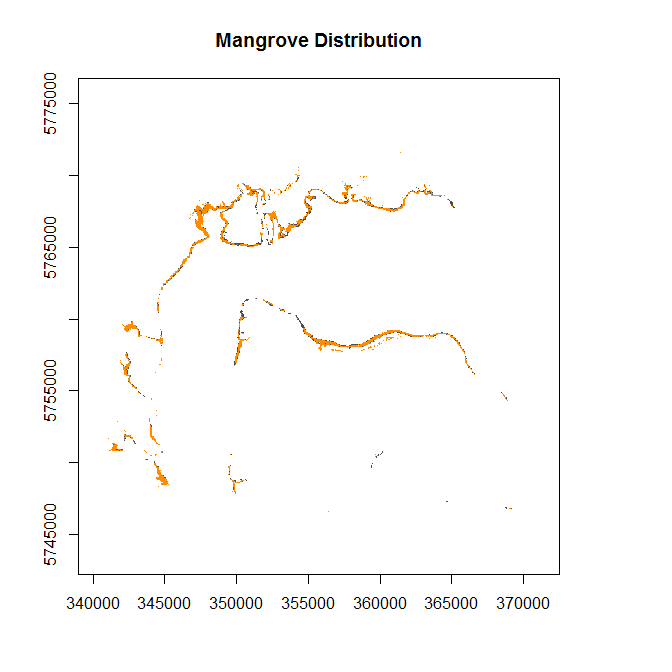We can see that there has been some change in mangrove cover, although the losses appear to be relatively minor.

At this stage it is important to check that the data are georectified (in the right location on Earth). This can be acheived by simply plotting the map and checking the coordinates. Otherwise, check the distribution maps against satellite images, perhaps by using packages such as ggmap, plotgooglemaps, googleVis etc. It is also important to check that the data are suitable for the task at hand.

## 3. Basic information for the data

We now use some of features of redlistr to get some basic information on the ecosystem distribution. However, before proceeding it is necessary to know the data by investigating their resolution (grain size), extent and spatial properties. Specifically, the coordinate reference system (CRS) of the datasets must be measured in metres, and is consistent to compare area.

isLonLat(mangrove.2000) 
##  FALSE
isLonLat(mangrove.2000)
##  FALSE
# If TRUE, they must be reprojected to a projected coordinate system

crs(mangrove.2000)@projargs == crs(mangrove.2017)@projargs
##  TRUE

Reprojection can be done by using the raster::projectRaster function.

We start by calculating the area of the dataset. Area is calculated here using the pixel count method, where the number of pixels classified as the ecosystem of interest is multiplied by the area of each pixel, giving the area of the ecosystem distribution. The area calculated is provided in square kilometres.

a.2000 <- getArea(mangrove.2000)
a.2000
##  13.6557
a.2017 <- getArea(mangrove.2017)
a.2017
##  12.3336

The results: at time 1 (2000), the ecosystem was 13.6557 km2, and at time 2, (2017) the ecosystem was 12.3336 km2.

### Creating binary ecosystem raster

An additional parameter in getArea is to specify which class to count if your raster has more than one value (multiple ecosystem data stored within a single file). However, for further functions, it may be wise to convert your raster into a binary format, containing only information for your target ecosystem.

First, if you do not know the value which represents your target ecosystem, you can plot the ecosystem out, and use the raster::click function. Once you know the value which represents your target ecosystem, you can create a new raster object including only that value. Here’s an example with a dummy raster.

# Create dummy raster for example
r <- raster(nrows=10, ncols=10)
r.multiple <- r
values(r.multiple) <- rep(c(1:10), 10)

# If the target ecosystem is represented by value = 5
r.bin <- r.multiple == 5 # Has values of 1 and 0
# Convert 0s to NAs
values(r.bin)[values(r.bin) != 1] <- NA

## 4. Assessing Criterion A

The IUCN Red List of Ecosystems criterion A requires estimates of the magnitude of change over time. This is typically calculated over a 50 year time period (past, present or future) or against a historical baseline (Bland et al., 2016). The first step towards acheiving this change estimate over a fixed time frame is to assess the amount of change observed in your data.

### Area change

Area change can be calculated using the getAreaLoss function. It simply calculates the difference in area between two datasets. It can accept data from numbers, RasterLayers or SpatialPolygons. Make sure to use area measure in km2 if you are using numbers as the input.

area.lost <- getAreaLoss(a.2000, a.2017)
# getAreaLoss(mangrove.2000, mangrove.2017) generates identical results

### Rate of change

In the Red List of Ecosystems, two methods are suggested to determine the rate of decline of an ecosystem, each of which assumes a different functional form of the decline (Bland et al., 2016). The proportional rate of decline (PRD) is a fraction of the previous year’s remaining area, while the absolute rate of decline (ARD) is a constant fraction of the area of the ecosystem at the beginning of the decline (Bland et al., 2016). These rates of decline allow the use of two or more data points to extrapolate to the full 50 year timeframe required in an assessment.

The annual rate of change (ARC) uses a compound interest law to determine the instantaneous rate of change (Puyravaud 2003).

To estimate the rate of changing using these methods, we use the getDeclineStats function.

decline.stats <- getDeclineStats(a.2000, a.2017, 2000, 2017,
methods = c('ARD', 'PRD', 'ARC'))
decline.stats
##   absolute.loss        ARD       PRD        ARC
## 1        1.3221 0.07777059 0.5972082 -0.5989987

Each method represent a different shape of decline. For further information about the choice of each of these methods to extrapolate refer to the IUCN Red List of Ecosystems guidelines (Bland et al., 2016).

Now, it is possible to extrapolate, using only two estimates of an ecosystems’ area, to the full 50 year period required for a Red List of Ecosystems assessment.

extrapolated.area <- futureAreaEstimate(a.2000, year.t1 = 2000,
ARD = decline.stats$ARD, PRD = decline.stats$PRD,
ARC = decline.stats$ARC, nYears = 50) ## Warning: 'futureAreaEstimate' is deprecated. ## Use 'extrapolateEstimate' instead. ## See help("Deprecated") and help("redlistr-deprecated"). extrapolated.area ## forecast.year A.ARD.t3 A.PRD.t3 A.ARC.t3 ## 1 2050 9.767171 10.12146 10.12146 50 years from our first estimate is the year 2050. As we included all three methods of calculating rate of decline currently included in the package, the results produced shows three estimated areas under the various decline scenarios. It is important to note that this relatively simple exercise is founded on assumptions that should be fully understood before submitting your ecosystem assessment to the IUCN Red List of Ecosystems Committee for Scientific Standards. Furthermore, the guidelines suggest using area estimates from more than two time points to estimate change, and providing a measure of uncertainty if possible. Please see the guidelines (Bland et al., 2016) for more information. If we were to use the Proportional Rate of Decline (PRD) for our example assessment, our results here will be suitiable for criterion A2b (Any 50 year period), and the percent loss of area is: predicted.percent.loss <- (extrapolated.area$A.PRD.t3 - a.2000)/a.2000 * 100
predicted.percent.loss
##  -25.88108

## 4. Assessing Criterion B (distribution size)

Criterion B utilizes measures of the geographic distribution of an ecosystem type to identify ecosystems that are at risk from catastrophic disturbances. This is done using two standardized metrics: the extent of occurrence (EOO) and the area of occupancy (AOO) (Gaston and Fuller, (2009), Keith et al., (2013)). It must be emphasised that EOO and AOO are not used to estimate the mapped area of an ecosystem like the methods we used in Criterion A; they are simply spatial metrics that allow us to standardise an estimate of risk due to spatially explicit catastrophes (Murray et al., (2017). Thus, it is critical that these measures are used consistently across all assessments, and the use of non-standard measures invalidates comparison against the thresholds. Please refer to the guidelines (Bland et al., 2016) for more information on AOO and EOO.

### Subcriterion B1 (calculating EOO)

For subcriterion B1, we will need to calculate the extent of occurrence (EOO) of our data. We begin by creating the minimum convex polygon enclosing all occurrences of our focal ecosystem.

EOO.polygon <- makeEOO(mangrove.2017)
plot(EOO.polygon)
plot(mangrove.2017, add = T, col = "green", legend = FALSE)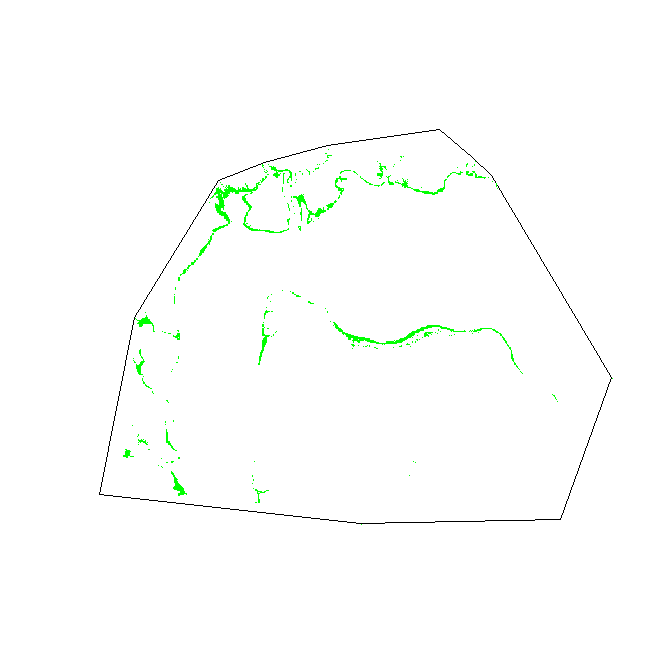We can then calculate the area of the EOO polygon.

EOO.area <- getAreaEOO(EOO.polygon)
EOO.area
##  608.8275

The calculated EOO area for this subset of mangroves in Victoria is 608.8275 km2. This result can then be combined with additional information required under B1(a-c) to assess the ecosystem under subcriteria B1.

### Subcriterion B2 (calculating AOO)

For subcriterion B2, we will need to calculate the number of 10x10 km grid cells occupied by our distribution. We begin by creating the appopriate grid cells.

AOO.grid <- makeAOOGrid(mangrove.2017, grid.size = 10000,
min.percent.rule = F)
plot(AOO.grid)
plot(mangrove.2017, add = T, col = "green", legend = FALSE)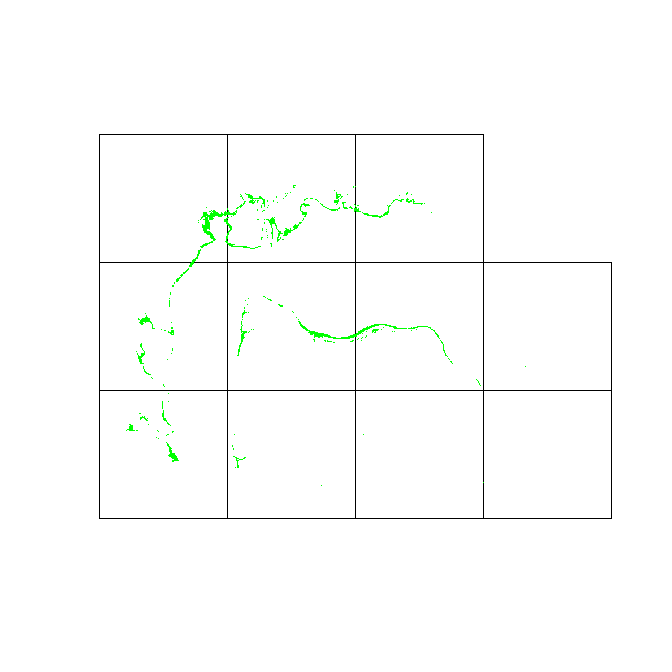Finally, we can use the created grid to calculate the AOO for our mangroves.

n.AOO <- length(AOO.grid)

# the getAOO function can also be used to directly get the AOO
# n.AOO <- getAOO(mangrove.2017, grid.size = 10000,
#                 min.percent.rule = T, percent = 0.1)
n.AOO
##  11

#### Grid uncertainty functions

Although we have a number for AOO 11, this may not be the actual minimum AOO for this ecosystem, because the placement of the AOO grid will influence this number. To take this into account, we have included a few additional functions. We will be using gridUncertainty in this vignette.

gridUncertainty simply moves the AOO grid systematically (with a small random movement around fixed points), and continues searching for a minimum AOO until additional shifts no longer produce improved results.

gU.results <- gridUncertainty(mangrove.2017, 10000,
n.AOO.improvement = 5,
min.percent.rule = F)
# If it takes your computer very long to run this command, consider reducing
# n.AOO.improvement

gU.results$min.AOO.df ## n.splits min.AOO ## 1 1 10 ## 2 2 10 ## 3 3 10 ## 4 4 9 ## 5 5 10 ## 6 6 10 ## 7 7 10 ## 8 8 9 ## 9 9 10 The result here shows that the minimum AOO does indeed change in response to where the AOO grid is placed, and that our original AOO value of 11 is not the true minimum AOO. The true minimum AOO (and the one which should be used for the assessment) is 9. It is also possible to plot out the grid which was used to produce the minimum AOO result, which can be used to report our final results. plot(gU.results$min.AOO.grid\$out.grid)
plot(mangrove.2017, add = T, col = "green", legend = FALSE)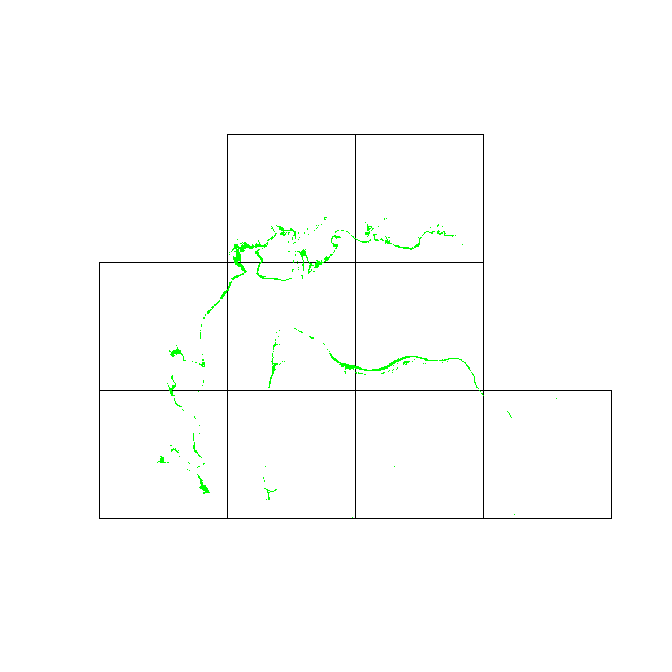#### Exporting results

The results of the above plot from gridUncertainty is a shapefile, which can be exported with the raster::shapefile function.

#### One percent rule

In addition to the size of the grids used (which will be different for species assessments), there is also an option in the makeAOOGrid function to specify whether a minimum of percent of the grid cell area must be occupied before they are included as an AOO grid. Typically, this is only used in very special cases for ecosystems with highly skewed distribution of patch sizes (Bland et al., 2016).

Here, we demonstrate the differences between including, or not including the one percent rule:

AOO.grid.one.percent <- makeAOOGrid(mangrove.2017, grid.size = 10000,
min.percent.rule = T, percent = 1)
plot(AOO.grid.one.percent)
plot(mangrove.2017, add = T, col = "green", legend = FALSE)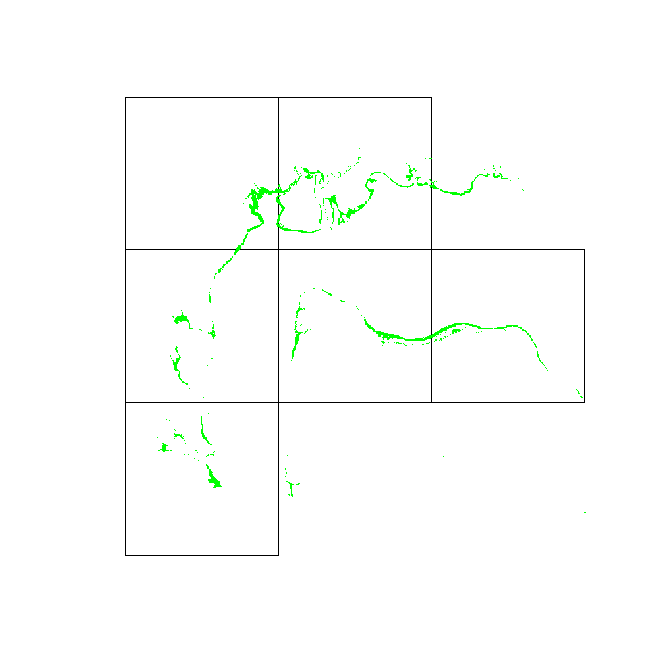There is an additional parameter - percent - which adjusts the threshold for the AOO grids. Here, we set it to 0.1% to demonstrate its functionalities.

AOO.grid.min.percent <- makeAOOGrid(mangrove.2017, grid.size = 10000,
min.percent.rule = T, percent = 0.1)
par(mfrow = c(2,2))
plot(AOO.grid, main = 'AOO grid without one percent rule')
plot(mangrove.2017, add = T, col = "green", legend = FALSE)
plot(AOO.grid.one.percent, main = 'AOO grid with one percent rule')
plot(mangrove.2017, add = T, col = "green", legend = FALSE)
plot(AOO.grid.min.percent, main = 'AOO grid with one percent rule at 0.1%')
plot(mangrove.2017, add = T, col = "green", legend = FALSE)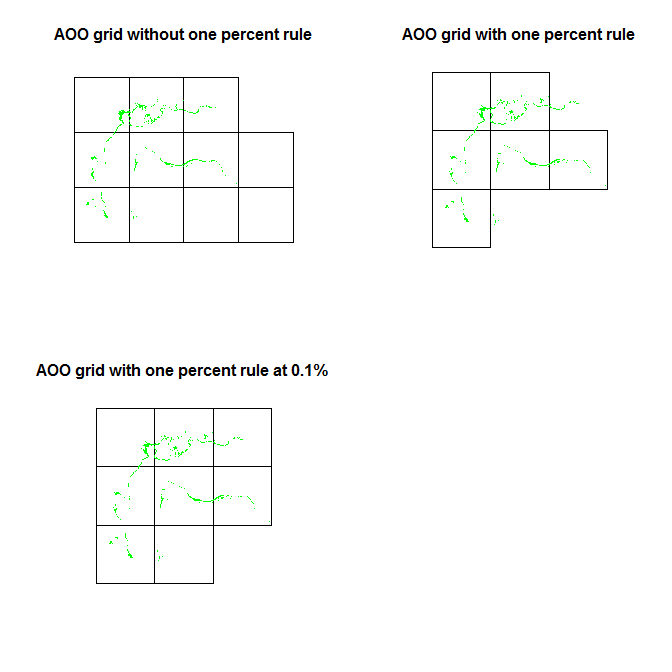## 5. Final words

We have demonstrated in this vignette a typical workflow for assessing an example ecosystem under the RLE. The methods outlined here can easily be adapted for the Red List of Threatened Species as well, with slight adjustments in the parameters (specifically the grid.size parameter for getAOO or gridUncertainty). We hope that with this package, we help ensure a consistent implementation for both red lists criteria, minimising any misclassification as a result of using different software and methods.